Documentation

sortClasses

Sort classes of confusion matrix chart

Description

example

sortClasses(cm,order) sorts the classes of the confusion matrix chart cm in the order specified by order. You can sort the classes in their natural order, by the values along the diagonal of the confusion matrix, or in fixed order that you specify.

Examples

collapse all

Create a confusion matrix chart and sort the classes of the chart according to the class-wise true positive rate (recall) or the class-wise positive predictive value (precision).

Load and inspect the arrhythmia data set.

isLabels = unique(Y);
nLabels = numel(isLabels)
nLabels = 13
tabulate(categorical(Y))
Value    Count   Percent
1      245     54.20%
2       44      9.73%
3       15      3.32%
4       15      3.32%
5       13      2.88%
6       25      5.53%
7        3      0.66%
8        2      0.44%
9        9      1.99%
10       50     11.06%
14        4      0.88%
15        5      1.11%
16       22      4.87%

The data contains 16 distinct labels that describe various degrees of arrhythmia, but the response (Y) includes only 13 distinct labels.

Train a classification tree and predict the resubstitution response of the tree.

Mdl = fitctree(X,Y);
predictedY = resubPredict(Mdl);

Create a confusion matrix chart from the true labels Y and the predicted labels predictedY. Specify 'RowSummary' as 'row-normalized' to display the true positive rates and false positive rates in the row summary. Also, specify 'ColumnSummary' as 'column-normalized' to display the positive predictive values and false discovery rates in the column summary.

fig = figure;
cm = confusionchart(Y,predictedY,'RowSummary','row-normalized','ColumnSummary','column-normalized');

Resize the container of the confusion chart so percentages appear in the row summary.

fig_Position = fig.Position;
fig_Position(3) = fig_Position(3)*1.5;
fig.Position = fig_Position;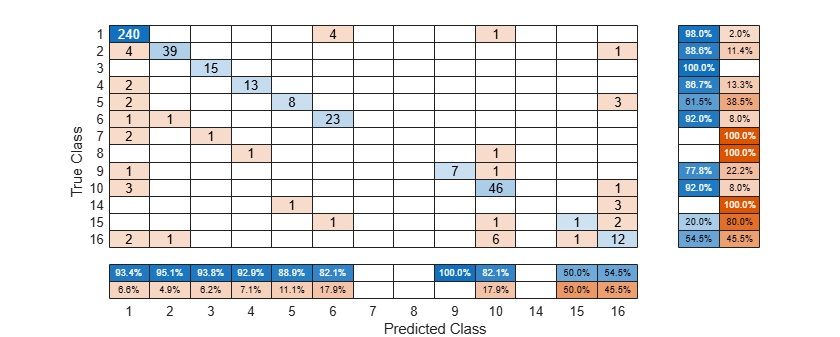To sort the confusion matrix according to the true positive rate, normalize the cell values across each row by setting the Normalization property to 'row-normalized' and then use sortClasses. After sorting, reset the Normalization property back to 'absolute' to display the total number of observations in each cell.

cm.Normalization = 'row-normalized';
sortClasses(cm,'descending-diagonal')
cm.Normalization = 'absolute';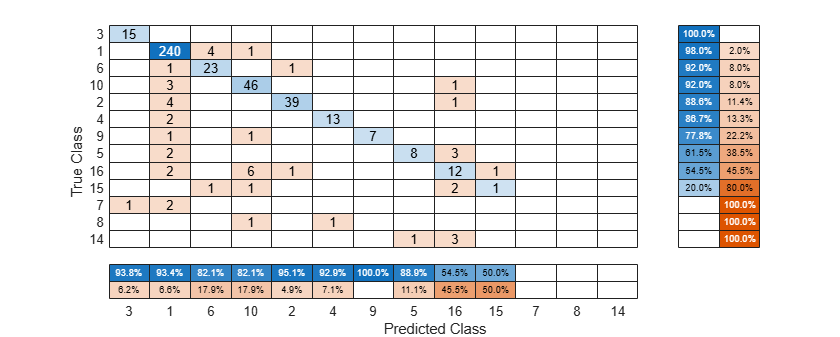To sort the confusion matrix according to the positive predictive value, normalize the cell values across each column by setting the Normalization property to 'column-normalized' and then use sortClasses. After sorting, reset the Normalization property back to 'absolute' to display the total number of observations in each cell.

cm.Normalization = 'column-normalized';
sortClasses(cm,'descending-diagonal')
cm.Normalization = 'absolute';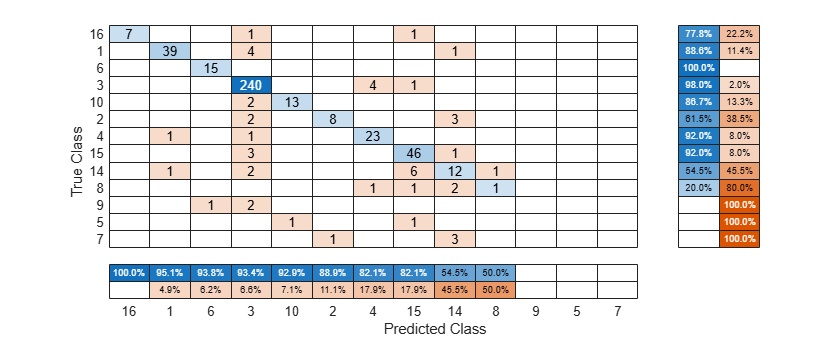Create a confusion matrix chart by using the confusionchart function, and sort the classes to cluster similar classes by using the 'cluster' option of the sortClasses function. This example also shows how to cluster by using the pdist, linkage, and optimalleaforder functions.

Generate a sample data set that contains eight distinct classes.

rng('default') % For reproducibility
trueLabels = randi(8,1000,1);
predictedLabels = trueLabels;

Insert confusion among classes {1,4,7}, {2,8}, and {5,6} for the first 200 samples.

rename = [4 8 3 7 6 5 1 2];
predictedLabels(1:100) = rename(predictedLabels(1:100));
rename = [7 8 3 1 6 5 4 2];
predictedLabels(101:200) = rename(predictedLabels(101:200));

Create a confusion matrix chart from the true labels trueLabels and the predicted labels predictedLabels.

figure
cm1 = confusionchart(trueLabels,predictedLabels);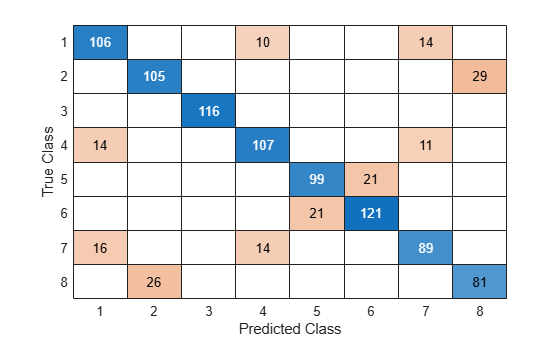Cluster Using 'cluster'

Sort the classes to cluster similar classes by using the 'cluster' option.

sortClasses(cm1,'cluster')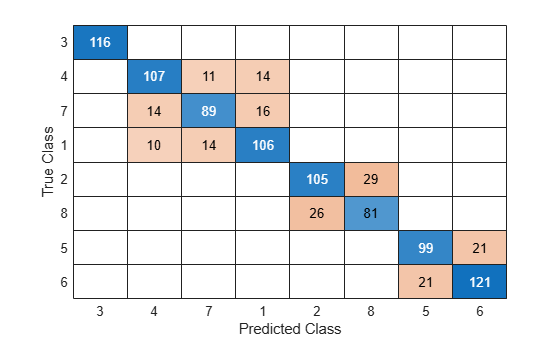Cluster Using pdist, linkage, and optimalleaforder

Instead of using the 'cluster' option, you can use the pdist, linkage, and optimalleaforder functions to cluster confusion matrix values. You can customize clustering by using the options of these functions. For details, see the corresponding function reference pages.

Suppose you have a confusion matrix and class labels.

m = confusionmat(trueLabels,predictedLabels);
labels = [1 2 3 4 5 6 7 8];

Compute the clustered matrix and find the corresponding class labels by using pdist, linkage, and optimalleaforder. The pdist function computes the Euclidean distance D between pairs of the confusion matrix values. The optimalleaforder function returns an optimal leaf ordering for the hierarchical binary cluster tree linkage(D) using the distance D.

D = pdist(m);
clusteredM = m(idx,idx);
clusteredLabels = labels(idx);

Create a confusion matrix chart using the clustered matrix and the corresponding class labels. Then, sort the classes using the class labels.

cm2 = confusionchart(clusteredM,clusteredLabels);
sortClasses(cm2,clusteredLabels)The sorted confusion matrix chart cm2, which you created by using pdist, linkage, and optimalleaforder, is identical to the sorted confusion matrix chart cm1, which you created by using the 'cluster' option.

Create a confusion matrix chart and sort the classes of the chart in a fixed order.

Load Fisher's iris data set.

X = meas([51:150,1:50],:);
Y = species([51:150,1:50],:);

X is a numeric matrix that contains four petal measurements for 150 irises. Y is a cell array of character vectors that contains the corresponding iris species.

Train a k-nearest neighbor (KNN) classifier, where the number of nearest neighbors in the predictors (k) is 5. A good practice is to standardize numeric predictor data.

Mdl = fitcknn(X,Y,'NumNeighbors',5,'Standardize',1);

Predict the labels of the training data.

predictedY = resubPredict(Mdl);

Create a confusion matrix chart from the true labels Y and the predicted labels predictedY.

cm = confusionchart(Y,predictedY);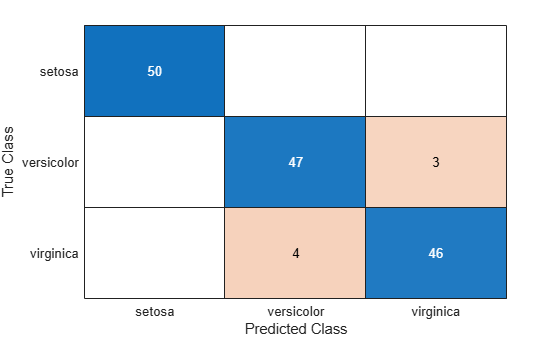By default, confusionchart sorts the classes into their natural order as defined by sort. In this example, the class labels are character vectors, so confusionchart sorts the classes alphabetically. Reorder the classes of the confusion matrix chart in a fixed order.

sortClasses(cm,["versicolor","setosa","virginica"])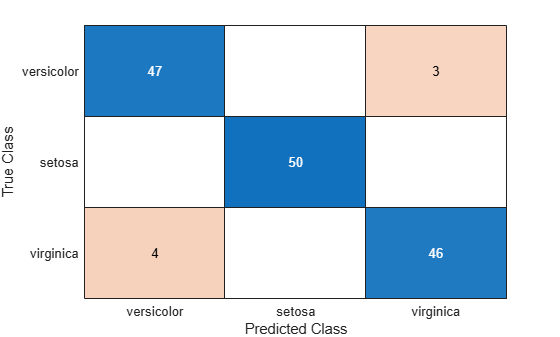Input Arguments

collapse all

Confusion matrix chart, specified as a ConfusionMatrixChart object. To create a confusion matrix chart, use confusionchart,

Order in which to sort the classes of the confusion matrix chart, specified as one of these values:

• 'auto' — Sorts the classes into their natural order as defined by the sort function. For example, if the class labels of the confusion matrix chart are a string vector, then sort alphabetically. If the class labels are an ordinal categorical vector, then use the order of the class labels.

• 'ascending-diagonal' — Sort the classes so that the values along the diagonal of the confusion matrix increase from top left to bottom right.

• 'descending-diagonal' — Sort the classes so that the values along the diagonal of the confusion matrix decrease from top left to bottom right.

• 'cluster' — Sort the classes to cluster similar classes. You can customize clustering by using the pdist, linkage, and optimalleaforder functions. For details, see Sort Classes to Cluster Similar Classes.

• Array — Sort the classes in a unique order specified by a categorical vector, numeric vector, string vector, character array, cell array of character vectors, or logical vector. The array must be a permutation of the ClassLabels property of the confusion matrix chart.

Example: sortClasses(cm,'ascending-diagonal')